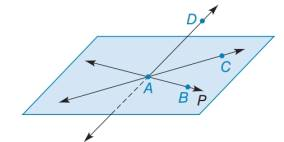Chapter 1.4, Problem 25E### Elementary Geometry for College St...

6th Edition
Daniel C. Alexander + 1 other
ISBN: 9781285195698

#### Solutions

Chapter
Section### Elementary Geometry for College St...

6th Edition
Daniel C. Alexander + 1 other
ISBN: 9781285195698
Textbook Problem
1 views

# Given: A B ↔ and A C ↔ in plane P as shown A D ↔ intersects P at points A ∠ C A B ≅ ∠ D A C ∠ D A C ≅ ∠ D A B What can you conclude?To determine

To describe:

The conclusion for given information.

Explanation

Given:

AB and AC lies on plane P as shown in figure below.

AD intersects P at points A.

CABDACDACDAB

The given figure is,

Calculation:

If the measure of two angles is equal, then the angles are known as congruent angles

### Still sussing out bartleby?

Check out a sample textbook solution.

See a sample solution

#### The Solution to Your Study Problems

Bartleby provides explanations to thousands of textbook problems written by our experts, many with advanced degrees!

Get Started